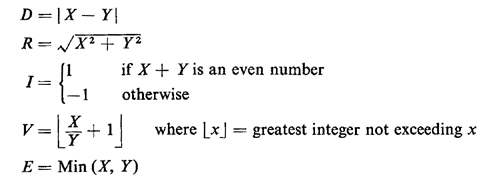# 2.6 FUNCTIONS OF RANDOM VARIABLES

In Example 2 it was sometimes convenient to discuss the sum of the two random variables, reflecting the total number of people on the minibus. This is a particular case of defining one or more random variables as functions of other random variables. This function can be any rule for assigning an experimental value to the new random variable(s), given the experimental value(s) of the original random variable(s). Given original random variables X and Y, several possible functions of X and Y are as follows:The derivation of probability laws for functions of random variables will be a primary concern of Chapter 3.